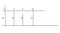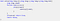# Problem Statement-

Lumberjack Mirko needs to chop down M metres of wood. It is an easy job for him since he has a nifty new woodcutting machine that can take down forests like wildfire. However, Mirko is only allowed to cut a single row of trees.

Mirko‟s machine works as follows: Mirko sets a height parameter H (in metres), and the machine raises a giant sawblade to that height and cuts off all tree parts higher than H (of course, trees not higher than H meters remain intact). Mirko then takes the parts that were cut off. For example, if the tree row contains trees with heights of 20, 15, 10, and 17 metres, and Mirko raises his sawblade to 15 metres, the remaining tree heights after cutting will be 15, 15, 10, and 15 metres, respectively, while Mirko will take 5 metres off the first tree and 2 metres off the fourth tree (7 metres of wood in total).

Mirko is ecologically minded, so he doesn‟t want to cut off more wood than necessary. That‟s why he wants to set his sawblade as high as possible. Help Mirko find the maximum integer height of the sawblade that still allows him to cut off at least M metres of wood.

The first line of input contains two space-separated positive integers, N (the number of trees, 1 ≤ N ≤ 1 000 000) and M (Mirko‟s required wood amount, 1 ≤ M ≤ 2 000 000 000).

The second line of input contains N space-separated positive integers less than 1 000 000 000, the heights of each tree (in metres). The sum of all heights will exceed M, thus Mirko will always be able to obtain the required amount of wood.

The first and only line of output must contain the required height setting.

Time limit:1s …. … . Source limit:50000B …. .. Memory limit:1536MB

# Discussion

So what we can conclude from above problem is we have to set some height from ground and from that height we have to cut every tree such that the sum of the cut off wood should be nearly same to the required amount of wood.

Let’s See the given example in problem statementHere we are cutting trees at height 15

So what approach comes to mind when we read this problem??

Yes ,Naive approach.

So we can directly take two nested for loop ,outer for setting height and inner loop for calculating sum of cutted wood of each tree.

But if we write code using this method then we will get TLE because required time limit is 1sec and our input range are large.

so we will use binary search here.

# Solution

We will use binary search on outer loop were we have to set the height.

we will take low=0 and high= max height of tree from tree set.

Now we will do same as general binary search where we take mid as (low+high)/2 and check for the sum of cutted wood .

If we get sum greater than or equal to required sum then we will make our low as mid+1 so that we can get best cutting height where we are not wasting too much wood.

If we get sum less than required sum then we will make our high as mid-1 so that we get sum atleast equal to required sum.

we will break if our low>high.function for calculating sum.

Thank you for reading this…….

Happy Learning :)

Pre Final year Computer Science and Engineering Student at RCOEM,Nagpur.

## More from Adesh Kotgirwar

Pre Final year Computer Science and Engineering Student at RCOEM,Nagpur.